Logarithms and Trigonometry in the HP 35's ROM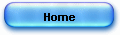_____Hand-held scientific calculators_____

Fast hardware based algorithms for
common transcendental functions

The secret of the algorithms

_________________________________________

You surely have been already struck by the speed of your hand-held scientific calculator computing the logarithm of a given number or the sine of an angle.

If your math is fresh enough, you possibly have guessed that the machine was using a sort of series approximation like Taylor or a polynomial approximation scheme.

In fact, the method used is very original regarding classical numerical calculus and is in perfect harmony with the hardware architecture of the machine.

Discovering these “hardware algorithms » is what the present article is about.

In 1972, the American Hewlett-Packard presented the world's first hand-held scientific calculators ever manufactured: HP 35. The name of this slide-rule killer was referring to its 3 x 5 in. keyboard on which was its 35 keys.

This marvelous object, that has all of the basic architecture of a micro computer was the result of combined evolution of micro electronics MOS/LSI circuits and of mastering micro programming so that the logic could embark fast transcendental operations (in a typical run time to less than a second).

The tool packed tightly 5 chips: three ROMs; a control and timing CT chip; and an arithmetic and register (AR) chip- for a total of 30,000 transistors.

The machine size was a severe constraint particularly implementing complex mathematical functions. In fact, as simple functions like 1/x or x2  can be easily realized using the for basic floating point operations, the case of transcendental and trigonometric functions seems to hit an insurmountable wall: execution time.

If your mathematic knowledge is not too blunt, you will remember of the convergence slowness of or an asymptotic representation or a series like the following (computed with Mathematica):

In =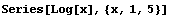Out =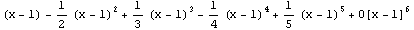And you will remark that –to obtain a good precision- a big number of terms must be computed. It’ll be the same thing with polynomial approximation.

But, if theses methods are usable of more important kind of machine, another constraint exists regarding hand help calculator architecture.

Due to the small size of the printed-circuit board, the calculator is organized in a digit-serial basis that saves connections on each 5 chips. So, the 3 busses are serial (Addresses IA, Instructions IS – and word select WS).

Each word is 56 bits long coding 14 binary-coded-decimal BCD digits. Mantissa is 10 BCD digits long, mantissa sign one long, exponent 2 digit long and exponent sign one digit long.

Display is done by 15 “7-segment-plus-decimal-point” LED. Each digit has a magnified spherical lend molded in the transparent plastic over it. The energy to drive the Led is pulsed using multiplexing digit then segment. An HP-made bipolar anode and cathode driver technology is used.

The careful reader remarked that with such an architecture the natural circuits for the arithmetic unit are adder-subtractor and shifter, the three main functions being adding, subtracting and shifting.

It should be noted that the floating point 4 operation (+ − ÷ *) algorithm is just using nothing more : mantissa division is done through subtraction and shift (pseudo division) while mantissa multiplication is using adding and shifting method (pseudo multiplication).

Thus, internal structure of the hand help calculator is particularly well suited for floating point calculus.

It could seems impossible to realize under these architectural constraints and with tiny ROMS, fast speed evaluation circuits for complex math (you press a key and you got immediately the sine of an angle).

That’s however what realized an American engineer Jack VOLDER –working for the firm CONVAIR to realize an airborne computer.

In 1956, the firm was involved in the development of the world’s first supersonic bomber for the US Air Force: Convair B-58 Hustler bomber that embarked a number of electronic technologies and, among them, a very accurate digital navigation devices using real-time trigonometric calculations.

Jack Volder, developed a method he named CORDIC (COordinate Rotation DIgital Computer) using only adders and shifters and that does not require a multiplier).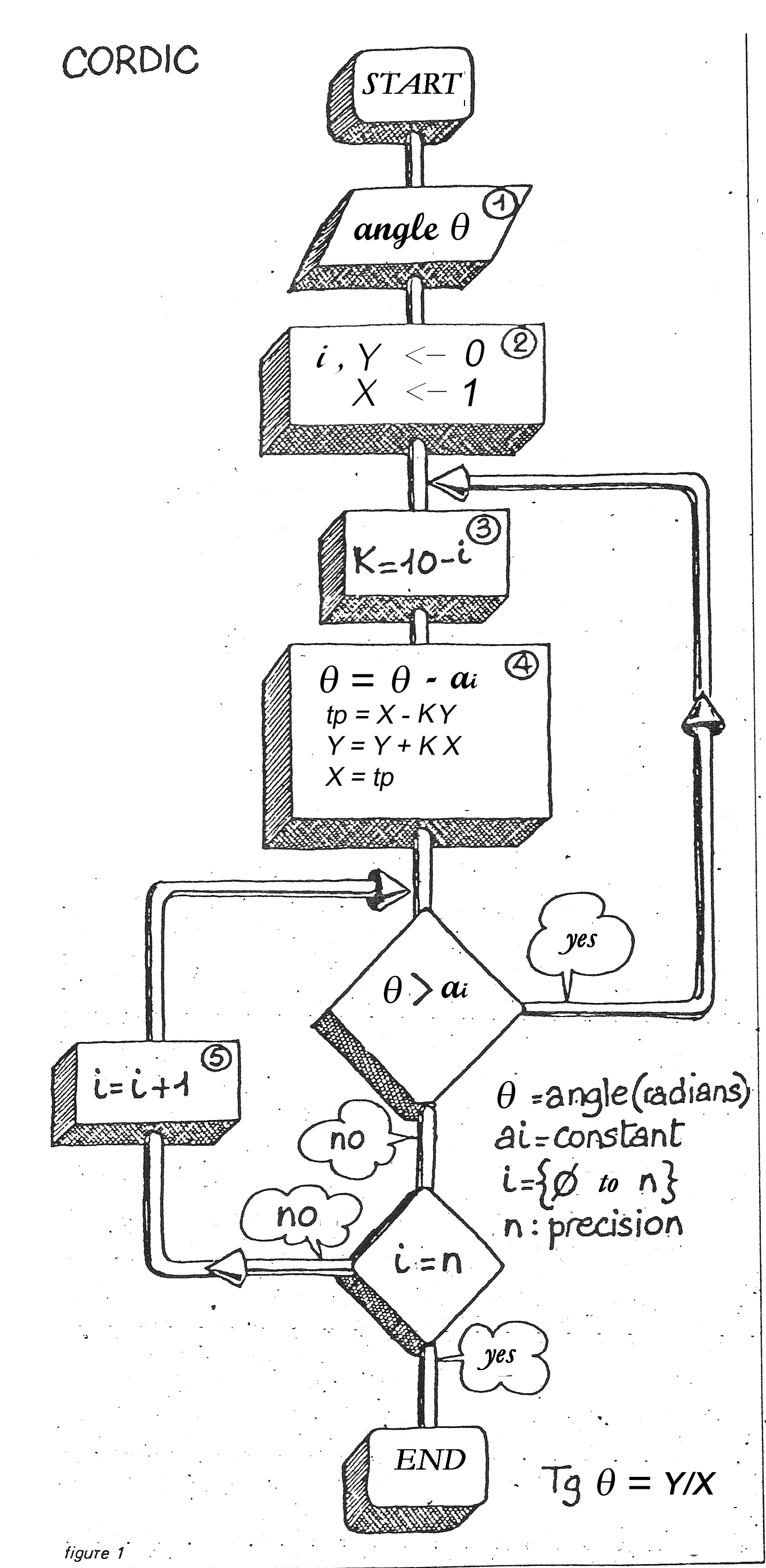Cordic is the name of the secret of the fast hardware-algorithm used by all hand held calculators starting with HP35, to compute log, sine and others.

The principle of the method is simple: to respect machine architecture, all operations are done using adder-subtracters and shifters.

In the case of the HP35, angles in degrees are converted to radians by multiplying by 2p /360.

By repeating 2p subtractions, argument can be set less then 2p.

The CORDIC result gives the tangent of the angle but sine and cosine, can be derived easily: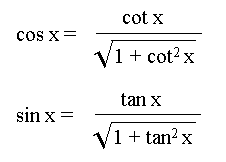An algorithm that fits the machine structure

The process consists to subtract –to the argument angle- constraints ai – stored in ROM, each one as many times as possible and to change the constant as soon as subtraction is no more possible.

Computations are arranged has shown in figure 1.

These constraints were named ATR (arc tangent radix) by Volder - (box 4, fig. 1).

Computing in BCD, ATR are:

a0 = tg-1 1 = arc tan (1) = π/4
a1 = tg-1 0.1 = 0.0996686
a2 = tg-1 0.01 = 0.0099996
a3 = tg-1 0.001 = 0.001
a4 = tg-1 0.0001 = 0.0001
and ai = 10-i for i great enough.

In box 4, fig. 1, angle subtraction (1rst line) was called “pseudo-division” by David S. Cochran HP35’s algorithm designer and micro programmer, and  computation of Xi and Yi: “pseudo-multiplication” (3 last lines in box) (following then Meggitt (cf. biblio)).

Studying closely the algorithm, you can remarks that it fits to the machine structure like a glove. This fact has played a miraculous role in the so fast development of the hand help calculator.

The trigonometric value is found in a few loops and in the typical time of 500 msec, on the HP35.

Of course, round-tripping works nicely, giving the angle from its tangent.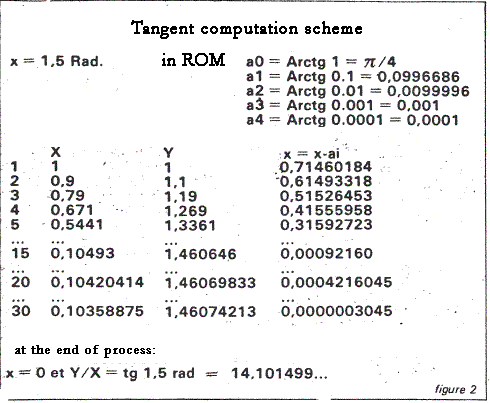Formal mathematical description of the algorithm can be found in the original article by Jack Volder.

But what is more astonishing, this method or similar can be extended (unified) to other type of transcendental computations like the research of the logarithm of a number.

An astonishing speed of convergence

The same convergence speed and architectural fit can also be found in the case of computation of the natural logarithm.

Like in trigonometric functions, we can always get back to a typical problem.

Thus, the case of natural logarithm is the only to consider. The common logarithm (base 10) can easily computed at he cost of a constant in ROM .

This value (ln 10) will be useful somewhere else in the rest of computation:

log x  = ln x/ln 10 and 10x = e x ln l0 where ln is the natural logarithm to the base e.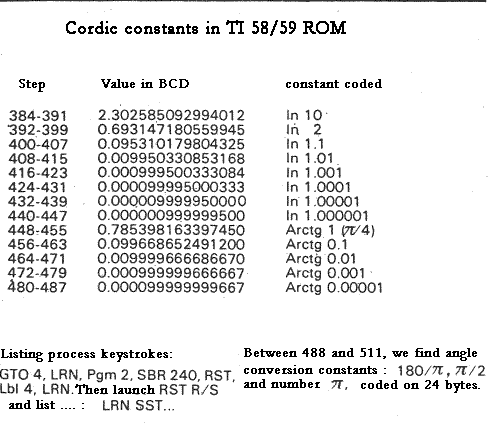We must take in account that, internally, numbers are represented in floating point format, what ever format is displayed.

So, for A=x×10P, we have the following relation:

ln A = ln x + p × ln 10 (with A>0).

We meet again constant ln 10.

Finally the only case to consider is the case of evaluation of the natural log of x with 1 ≤ x ≤ 10.

The following constants are stored in ROM.

ln 10
ln 2  = ln (1+100)
ln 1.1 = ln (1+10-1)
ln 1.01 = ln (1+10-2)
ln 1.001 = ln (1+10-3) etc. to the machine precision.

Figure 2 shows the « real » constants burnt in the ROM of the Texas TI 59 calculator.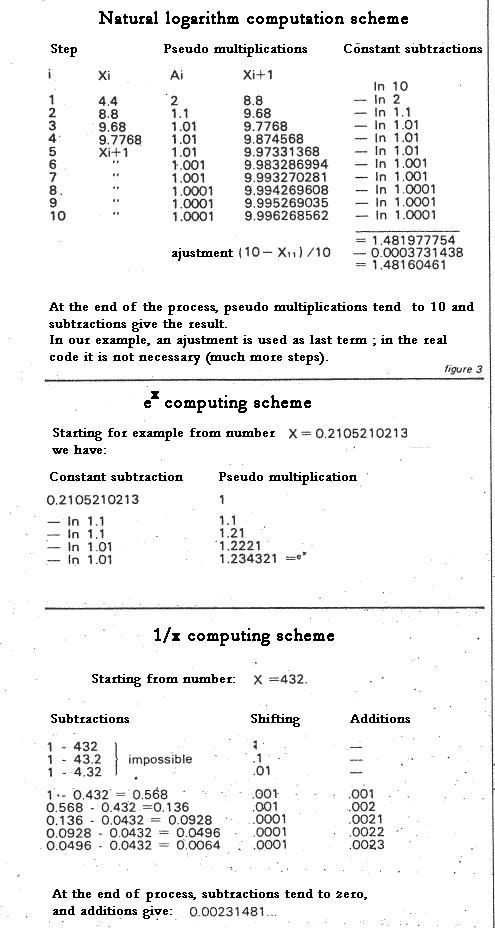In another article, I’ll comment in detail -line by line- the HP35 ROM micro program for ln x.

It uses exactly the same approach. I'll give also the execution trace of the real ROM.

Roughly, computing the ln x can be sketched as shown figure 3.

In each loop, the number Xi is multiplied by a value ai ; without the result exceeding 10.

Should this happens, ai constant is changed in decreasing order (2, 1.1, 1.01, 1.001 ...) etc.

In each loop, the subtraction of ln ai from ln 10 is done.

The careful reader remarked that all multiplications to be done can easily be replace by shifting (right) and adding, accordingly to the method principle.

Jack Volder's approach can be applied to the evaluation of many functions: x, tg n, ln x.

For our hand help calculator, other useful functions can be obtained by derived ways:  P->R, R-> P, yx (as being equal to e x ln y), etc.

Finally, and it is not the less surprising, a certain Briggs (way back in 1624!) has described a method in his “Arithmetica Logarithmica” that is close to Volder’s approach
(see on this site the article "Briggs and the HP35").

Hand held calculator does not exist yet! The problem was to help the labor of the first humans calculating numerical tables.

The next time you’ll hit the ln key of your beloved HP or Texas, maybe you’ll find amusing to thing that the machine is recalculating the log table like they did at the good old time of Briggs et Neper !

Jacques LAPORTE

Bibliography

J.E. VOLDER, “The Cordic Trigonometric Computing Technique”, IEEE Transactions on Electronic Computers, Sept. 1959,

The article of (1959) deserves an attentive reading. We give it here in PDF format for pedagogical purposes.

Volder’s approach has been generalized by
J.S. Walther for HP in 1971. In his article (PDF en download), the general algorithm is applied to number of other functions: Walther, J.S., “A unified algorithm for elementary functions,” Spring Joint Computer Conf., 1971, proc., pp379-385

Texas Instruments Software Exchange Newsletter » Vol II  n°2 et 3.

, Pseudo Division and Pseudo Multiplication processes, IBM Journal, Res & Dev, April 1062, 210-226 (in fact, it is the MEGGIT's approach of the Cordic which is implemented in the HP35's ROM).

In English, the Grant R. Griffin’s is very good.
But nothing is better first than reading original papers by VOLDER, WALTHER & MEGGITT.

___________________________________________________________________________________________________

PS Feb 2005.

This article was first published in the French review l’Ordinateur Individuel – n° 24 (February 1981).

English version Feb 2005.

It is the original text that I republish here (only a few typos have been corrected).

For the sake of pedagogy, I wrote once a ‘C’ language equivalent for the process shown figure 1. Compilation is straightforward: gcc

But one warning though: Cordic is not an algorithm to be implemented in whatever programming language: keep in mind it is a hardware architecture!

You can now prefer experimenting Cordic -step by step- on a simulator running the real HP35 ROM, see : The HP-35 Microcode Simulator

___________________________________________________________________________________________________

Ancient footprints

Volder wrote a first internal report about CORDIC in 1956 and finally published a final paper in the 1959 Proceedings of the Western Joint Computer Conference. Apparently, Volder quit Convair before the CORDIC prototype had been built. He put his own trigonometric prototype on foot with an associate named Macmillan. Both managed to put it under HP eyes (Bill Hewlett himself?) probably around summer 1965.

Impressed or not, the HP guys would remember the CORDIC algorithm when the firm initiated the project of its first scientific calculator: HP 9100A.

Osborne (freelance consultant) who designed almost all HP 9100’s hardware worked with David Cochran (HP employee) for the firmware.

That was Volder himself who introduced to Cochran “pseudo multiplication” and “pseudo division” studied by IBM’s J. E. Meggitt and published in 1962. In the 9100 micro program, Cochran used Volder’s algorithm for trigonometric functions and Meggitt’s for logarithms, exponentials, and square root.
Both approaches are very close: they use only "shift" and "add" operations.

The basic ingredients for brewing HP35 ROM were on the table.